# gggibbous: Moon charts, a pie chart alternative for two groups

## Moon charts and pie charts

A pie chart divides a circle into multiple sections where the arc lengths (and so also the areas) of the sections represent proportions of a whole. A moon chart, similarly, divides a circle into sections where the areas represent proportions of a whole, but in a moon chart the areas are drawn as crescent or gibbous portions of a circle—like the phases of the moon.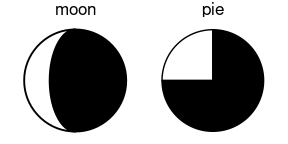The motivation behind using a moon chart instead of a pie chart is primarily one of aesthetic choice. Note also that because the sections of a moon chart are swept from one or the other side of the circle, they are generally only appropriate for depicting one or two groups.

Moon charts are similar to Kosara’s (2019)1 “circular slice” chart. In studying subjects’ perception of percentages in different chart types, the “circular slice” performed similarly to pie charts. Moon charts differ from the “circular slice” in that the latter slides a second disc of the same size over a base circle, more like a lunar eclipse than the phases of the moon. Both depend on area as the perceptual cue, however.

## gggibbous and its usage

gggibbous extends the ggplot2 data visualization package to provide support for moon charts in R. Unlike the pie charts supported natively by coord_polar() in R, moon charts in gggibbous do not require any special coordinate system. They are drawn most similarly to points in ggplot2: their position is defined by an x and a y coordinate and their size is defined independently of the coordinate system, so they always remain circular.

library(gggibbous)
#> Loading required package: ggplot2
ggplot(data.frame(x = 1:5, y = 1, size = 2^(0:4)), aes(x, y, size = size)) +
geom_moon() +
geom_point(y = 2) +
lims(x = c(0.5, 5.5), y = c(0.5, 2.5)) +
scale_size(range = c(5, 10))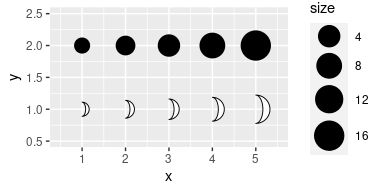Two new aesthetics are also important in geom_moon: ratio and right.

### The ratio aesthetic

ratio controls the proportion of the moon to be drawn. It must be between 0 (a “new moon” where nothing is actually drawn) and 1 (a “full moon”, i.e. a circle).

ggplot(data.frame(x = 1:5, y = 0, ratio = 0:4 * 0.25), aes(x = x, y = y)) +
geom_moon(aes(ratio = ratio), size = 20, fill = "black") +
geom_text(aes(y = y + 1, label = ratio)) +
lims(x = c(0.5, 5.5), y = c(-1, 1.4)) +
theme_void()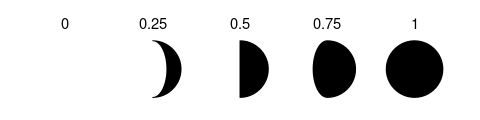### The right aesthetic

right takes a boolean value that controls whether the moon is “waxing” or “waning”—that is, whether it is “filled” from the right or the left.

One way to make a “complete” moon with two colors is to use right = TRUE for one color and right = FALSE for the other, with complementary ratios. (See the examples below for some other approaches.)

tidymoons <- data.frame(
x = rep(1:3, 6),
y = rep(rep(3:1, each = 3), 2),
ratio = c(1:9 / 10, 9:1 / 10),
right = rep(c(TRUE, FALSE), each = 9)
)

ggplot(tidymoons) +
geom_moon(aes(x, y, ratio = ratio, right = right, fill = right)) +
lims(x = c(0.5, 3.5), y = c(0.5, 3.5))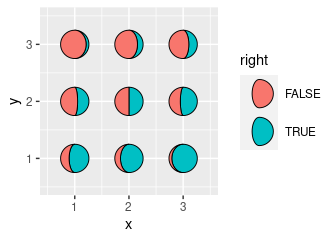## Legend key glyphs

gggibbous includes three key glyphs for different types of legends: draw_key_moon, draw_key_moon_left, and draw_key_full_moon

draw_key_moon—the default in geom_moon—draws a gibbous moon with right = TRUE (see above).

draw_key_moon_left draws a crescent moon from the left that is complementary to the gibbous moon in draw_key_moon, which is useful for combined legends:

ggplot(tidymoons, aes(x, y, ratio = ratio, right = right, size = 2^x)) +
geom_moon(data = subset(tidymoons, right), fill = "violetred") +
geom_moon(
data = subset(tidymoons, !right), fill = "turquoise3",
key_glyph = draw_key_moon_left
) +
lims(x = c(0.5, 3.5), y = c(0.5, 3.5)) +
scale_size("size", range = c(5, 10), breaks = 2^(1:3))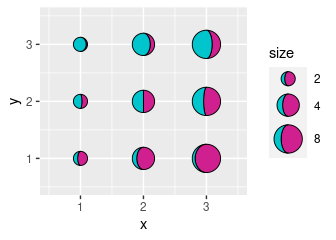draw_key_full_moon draws a circle. It is similar to the “point” key glyph, but the size is calculated slightly differently, so it is more appropriate if you want the size of legend moons and the size of the plot moons to match.

ggplot(tidymoons) +
geom_moon(
aes(x, y, ratio = ratio, right = right, fill = right, size = 2^x),
key_glyph = draw_key_full_moon
) +
lims(x = c(0.5, 3.5), y = c(0.5, 3.5)) +
scale_size("size", range = c(5, 10), breaks = 2^(1:3)) +
scale_fill_manual(values = c("firebrick1", "dodgerblue2")) +
theme(legend.box = "horizontal")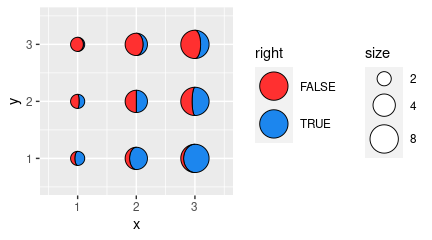## Worked examples

### Moon charts on maps

One common use of multiple pie charts is to represent proportions at different coordinates on a map. The x and y dimensions are already committed to the map coordinates, so proportional visualizations like bar charts are more difficult. This is a perfect opportunity to try out moon charts!

Pie chart maps are popular in population genetics, so let’s look at an example from that field. The dmeladh data2 contains frequencies of two variants of the Adh gene in Australian and Papua New Guinean fruit fly populations. Many of these populations are close together, so we have to deal with overplotting, which we do manually below.

dmeladh_adj <- dmeladh
dmeladh_adj$long <- dmeladh$Longitude + c(
-2, 0, -2, 2, -3, 3, 3, 2, 3, 4, -2.5, -2.5, -1, -2, -2.5, -4, 2.5,
5, 6, 7, 2, -7, -5.5, -3, 0, -7, -2, 3, 5.5, 0.5, -1, -1.5, -3, 2)
dmeladh_adj$lat <- dmeladh$Latitude + c(
-2, 2, 0, 1, 0, 0, 0, 2, 0.5, -1, 1, -1.5, 2, 4, 1.5, 0, 2,
1, -1, -3, -2, 1, -1, -2, -3, -2, -4, -3, -1, 1.5, 2, 2, -2, 0)

geom_polygon(
data = map_data(
"world", region = "(Australia)|(Indonesia)|(Papua New Guinea)"),
aes(group = group),
fill = "gray80"
) +
geom_segment(aes(xend = Longitude, yend = Latitude), color = "gray20") +
geom_point(aes(Longitude, Latitude), size = 0.75, color = "gray20") +
scale_size(range = c(4, 10)) +
coord_map(xlim = c(110, 160), ylim = c(-45, -5)) +
theme_void() +
theme(
legend.position = c(0.05, 0.05),
legend.direction = "horizontal",
legend.justification = c(0, 0)
)

moonmap +
geom_moon(
aes(ratio = AdhS / 100, size = N),
right = FALSE, fill = "gold", color = "gold",
key_glyph = draw_key_moon_left
) +
geom_moon(
aes(ratio = AdhF / 100, size = N),
fill = "forestgreen", color = "forestgreen"
)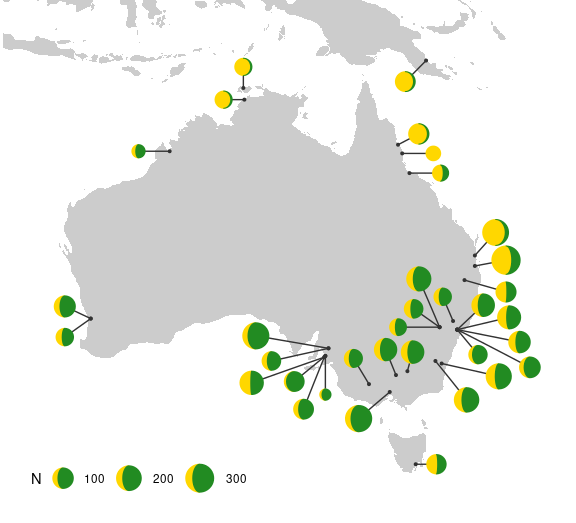If we want to label the alleles in the legend explicitly, then we need to map them to a group, which requires that we rearrange the data into a “longer” (“tidy”) format.

tidyadh <- reshape(
v.names = "percent",
timevar = "allele",
idvar = c("Locality", "Latitude", "Longitude", "long", "lat", "N"),
direction = "long"
)
tidyadh$right <- rep(c(TRUE, FALSE), each = nrow(dmeladh_adj)) moonmap + geom_moon( data = tidyadh, key_glyph = draw_key_full_moon, aes(ratio = percent / 100, fill = allele, color = allele, right = right, size = N) ) + scale_fill_manual(values = c("forestgreen", "gold")) + scale_color_manual(values = c("forestgreen", "gold"))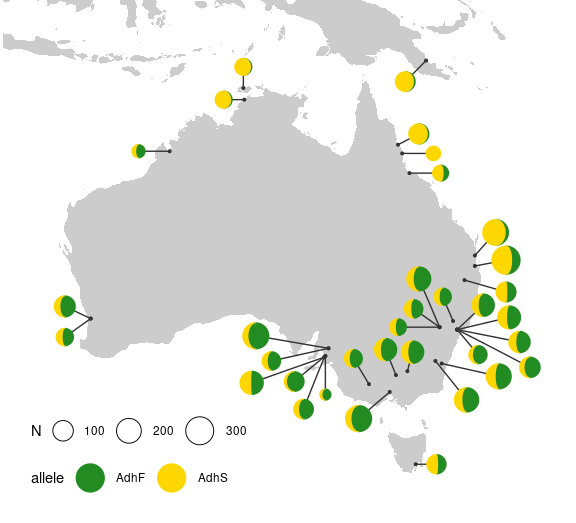### Lunar data Sometimes you just want to plot literal representations of the moon. The lunardist data, adapted from NASA, contains the distance from the Earth to the Moon for each day in 2019, as well as the dates (in UTC) of each occurrence of the four principal phases of the moon. We can plot those principal phases using moon charts (which in this case are identical to pie charts). moonphase <- subset(lunardist, !is.na(phase)) moonphase$percent <- ifelse(
moonphase$phase == "new", 0, ifelse(moonphase$phase == "full", 1, 0.5))

ggplot(lunardist, aes(date, distance)) +
geom_line() +
# Plotting the lower layer as a full circle also works in most cases
geom_moon(data = moonphase, ratio = 1, size = 5, fill = "black") +
geom_moon(
data = moonphase, aes(ratio = percent),
size = 5, fill = "yellow", right = moonphase\$phase == "first quarter"
)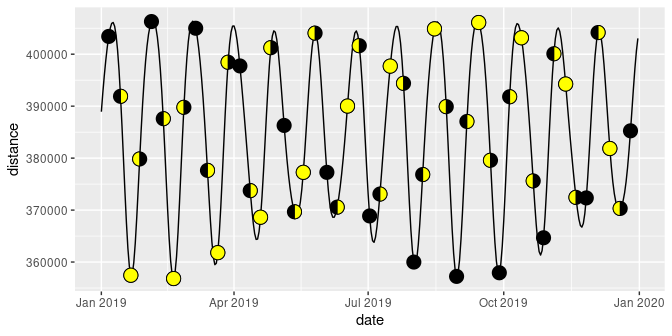### Harvey balls

“Harvey balls” are essentially pie charts used for qualitative comparisons, often in tabular format. We can use moon charts for the same purpose.

First, let’s make up some data:

rest_names <- c(
"Anscombe's Luncheonette", "Chai Squared", "Tukey's Honest Southern Diner",
"Bagels ANOVA", "Spearmint Row"
)
restaurants <- data.frame(
Restaurant = factor(rest_names, levels = rest_names),
Food = c(5, 3, 4, 4, 1),
Decor = c(2, 5, 3, 1, 5),
Service = c(4, 2, 3, 3, 5),
Price = c(4, 5, 2, 5, 2)
)

As a regular table:

Restaurant Food Decor Service Price
Anscombe’s Luncheonette 5 2 4 4
Chai Squared 3 5 2 5
Tukey’s Honest Southern Diner 4 3 3 2
Bagels ANOVA 4 1 3 5
Spearmint Row 1 5 5 2

And now as a table with Harvey moons:

# First we reshape the data into "long" format to facilitate plotting
rest_cats <- c("Food", "Decor", "Service", "Price")
tidyrest <- reshape(
restaurants,
varying = rest_cats,
v.names = "Score",
timevar = "Category",
times = factor(rest_cats, levels = rest_cats),
idvar = "Restaurant",
direction = "long"
)

ggplot(tidyrest, aes(0, 0)) +
geom_moon(aes(ratio = (Score - 1) / 4), fill = "black") +
geom_moon(aes(ratio = 1 - (Score - 1) / 4), right = FALSE) +
facet_grid(Restaurant ~ Category, switch = "y") +
theme_minimal() +
theme(
panel.grid = element_blank(),
strip.text.y.left = element_text(angle = 0, hjust = 1),
axis.text = element_blank(),
axis.title = element_blank()
)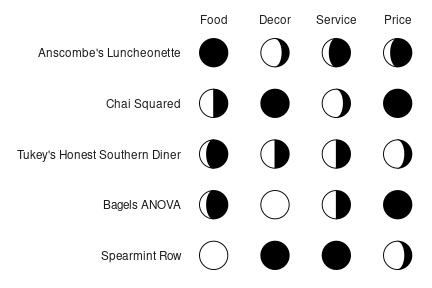1. Kosara, R. 2019. Circular Part-to-Whole Charts Using the Area Visual Cue. EuroVis 2019 - Short Papers. https://doi.org/10.2312/evs.20191163↩︎

2. Oakeshott, J.G., et al. 1982. Alcohol dehydrogenase and glycerol-3-phosphate dehydrogenase clines in Drosophila melanogaster on different continents. Evolution, 36(1): 86-96.↩︎